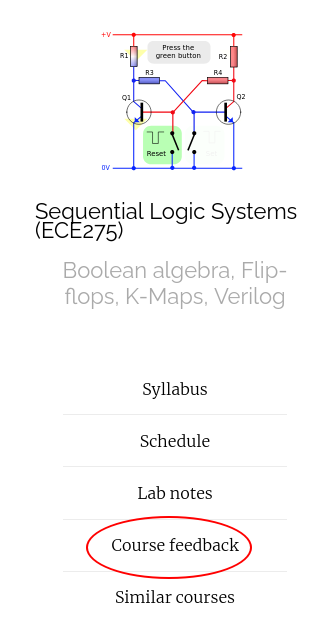## Announcments

• Midterm is on Oct 6th, 9 AM in class.
• Homework 4 is due midnight.

## Functional decomposition takeaways

• Functional decomposition allows us to trade-off Fan-in with Gate cost. Sometimes it also reduces the gate cost.
• Even modern CAD algorithm's donot try all possible functional decompositions. They use "rules of thumb" or heuristics. You will not be penalized for not able to find the lowest cost decomposition.

## Office hours review

• All two variable expressions can be reduced two a single gate.
• When a problem says that inputs are only available in "uncomplemented" form, then it means that if you take an inversion of input, the cost of NOT gate will be counted.

## Signed number system

• Sign and Magnitude representation
• 1's complement = $$-m = 2^N - 1 - m$$ = Flip each bit.
• 2's complement = $$-m = 2^N - m$$ = Add 1 to Ones' complement.
DecimalSM1's comp2's comp
-4- - 100
-3111 100 101
-2110 101 110
-1101 110 111
-0100 111 000
0000 000 000
1001 001 001
2010 010 010
3011 011 011

## 2's complement shortcut

Find the 2's complement of 01001100.

Decimal1's comp2's comp
-4 - 100
-3 100 101
-2 101 110
-1 110 111
-0 111 000
0 000 000
1 001 001
2 010 010
3 011 011

Decimal1's comp2's comp
-4 - 100
-3 100 101
-2 101 110
-1 110 111
-0 111 000
0 000 000
1 001 001
2 010 010
3 011 011

## Design a 3-bit 1's complement circuit

that complements a 3-bit signed magnitude representation $$x_2 x_1 x_0$$

Design a Multi-output circuit that adds two 1-bit binary numbers $$x_0$$ and $$y_0$$ and outputs a sum and carry bit. $s_0 = x_0 \boxplus y_0$ $c_0 = ( x_0 \boxplus y_0 ) \% 2$

Design a Multi-output circuit that adds three 1-bit binary numbers $$x_1$$, $$y_1$$ and $$c_0$$ outputs a sum and carry bit. $s_1 = x_1 \boxplus y_1 \boxplus c_0$ $c_1 = ( x_1 \boxplus y_1 ) \% 2$

that complements a 3-bit signed magnitude representation $$x_2 x_1 x_0$$
https://vikasdhiman.info/ECE275-Sequential-Logic/